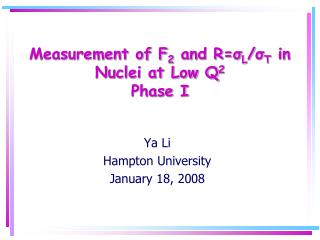# Measurement of F 2 and R= σ L / σ T in Nuclei at Low Q 2 Phase I - PowerPoint PPT PresentationDownload PresentationMeasurement of F 2 and R= σ L / σ T in Nuclei at Low Q 2 Phase I

Measurement of F 2 and R= σ L / σ T in Nuclei at Low Q 2 Phase I
Download Presentation## Measurement of F 2 and R= σ L / σ T in Nuclei at Low Q 2 Phase I

- - - - - - - - - - - - - - - - - - - - - - - - - - - E N D - - - - - - - - - - - - - - - - - - - - - - - - - - -
##### Presentation Transcript

1. Measurement of F2 and R=σL/σT in Nuclei at Low Q2Phase I Ya Li Hampton University January 18, 2008

2. Outline • Physics Overview • Physical Motivation and Description of Experiments E02-109/E04-001 (Jan05) • Analysis Status • Preliminary Results • Future Plans

3. e(E) e’(E’) θ Q2 N Transverse virtual photon flux Virtual photon polarization parameter e - N scattering One-Photon-exchange Approximation σT (σL) is the Transverse (Longitudinal) virtual photon Cross Section Mp- mass of the Proton W– invariant mass Q2 - Negative squared mass of the virtual photon

4. Reduced Cross-section L/T separations - Rosenbluth Method At ε =1,  F2 Diff.  FL { At ε =0,  F1 Fit reduced cross section linearly withεat fixed W2 and Q2 (or x, Q2) --> Need multiple beam energies. • Linear fit yields: σL = Slope σT = Intercept

5. Physical Motivation • Sparse data available in Resonance Region on Fundamental Separated Structure Functions in Nuclei (F1,F2,FL, R) • Low Q2 L/T Structure Function Moments • Study Quark-Hadron Duality in Deuteron, Neutron, and Nuclei. • Also, important input for Spin Structure Function extraction from asymmetry measurements, RCs, etc…

6. Motivation from Neutrino Experiments • New generation of neutrino experiment are being built to investigate neutrino oscillations and interactions -i.e. MinervA, mini-Boone, MINOS, , T2K • Input for neutrino cross section models, needed for new generation of oscillation experiments around the world • However…Neutrino Cross Sections still poorly understood • Neutrino Oscillations Dm2 ~ E / L, requires E in few GeV range (same as JLab!) • Global models needed linking electron and neutrino scattering data Resonance region is a major contribution!

7. Experiment Description • E02-109: Meas. of F2 and R on Deuterium. • E04-001: Meas. of F2 and R on Carbon, Iron, and Aluminum. Also, Hydrogen for crosschecks. (Data from this will also be used by neutrino scattering community). • Beam Energies used were: 4.6, 3.5, 2.3, and 1.2 GeV. • Experiments ran for ~2 weeks in Hall C of January 2005 to cover 0.05 < Q2 < 2 (GeV)2 and 0.5 <W2 < 4.25 (GeV)2.

8. Experiment setup and procedure • Jlab Hall C • HMS for scattered electrons • SOS for positrons At fixed Ebeam, θc, scan E’ from elastic to DIS. Repeat for each Ebeam, θcto reach a range in ε for each W2, Q2. HMS SOS

9. Kinematics' Coverage • Rosenbluth Separation Data • Targets: D, C, Al, Fe , and some H • Final Uncertainties estimated at ~1.6 % pt-pt in e (2% normalization). • Low Q2 data for n modeling • Targets: H,D, C, Al • Final Uncertainties estimatedat ~3 - 8% (Much larger RCs and rates) Rosenbluth separations at multi. energies

10. HMS Momentum 2.75 GeV 2.36 GeV 2.00 GeV 1.75 GeV Analysis Methodology • Bin efficiency corrected e- yield in p/p - (∆p/p = +/- 8%, ∆  = +/- 35 mrad) • Subtract scaled dummy yield bin-by-bin, to remove e- background from cryogenic target Aluminium walls. • Subtract charge-symmetric background from π0 decay via measuring e+ yields. • Apply acceptance correction for each - bin. • Apply radiative corrections bin-by-bin. • Apply  bin-centering correction and average over  => for each  bin.

11. Structure Function Extraction • Rosenbluth separations at each W2 and Q2 where possible (range in ε exist to perform a good linear fit) • A global fitting of F2 and R over the entire kinematics range.

12. Analysis Status Completed Completed Completed Completed Completed Completed Completed for E’ > 1.5 GeV Completed Completed Completed Completed Completed Preliminary Sieve Slit Completed Nearly completed inelastic ~5% and Preliminary QE Detector Calibrations Calorimeter Efficiency Cerenkov Efficiency Tracking Efficiency Trigger Efficiency Computer Dead Time Acceptance Corrections Beam Position Offsets Beam Position Stability Kinematics Offsets Beam Energy Stability Study Target Density Corrections Optics Checks Radiation Corrections Charge Symmetric Background Cross-Sections

13. Beam and Targets Position Offsets Where ∆X is the offset of the beam, ∆Z is the offset of the target relative to the pivot, and θ is the HMS angle. From geometry, we can express this as:

14. Beam and Targets Position Offsets • Comparing the beam position of the Data to the Monte Carlo for different , we’ve arrived at these offsets (mm) FeCHD ∆X = 0.8627 1.1837 1.0795 1.1420 Err = 0.2811 0.4530 0.3043 0.3501 ∆z = 2.5009 -2.0983 1.7682 1.6016 Err = 0.6245 0.4530 0.4340 0.4058

15. Beam and Targets Position Offsets Data and MC (before position corrections) Data and MC (after position corrections)

16. Subtract off Charge Symmetric electrons by subtracting off positron Cross-Sections. e+ γ e- π0 e+ γ e- Polynomial Fit across Theta Charge Symmetric Backgrounds Parameterized e+ CS SOS e+ Cross-section HMS e+ Cross-section

17. Preliminary Cross Sections

18. Preliminary Cross Sections • Model only accounts for Inelastic Cross Section • Results do not account for Quasi-Elastic contribution • Model does not accurately account for resonances at low Q2

19. Plans in the Future • Extract position cross sections for CSB correction • Extract QE and Inelastic cross sections • Improve on Global Fits of Data • Complete Final Cross Sections • Rosenbluth Separations • Extract Structure Functions